#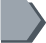Math word problems for kids - Easy math word problems for kids with answers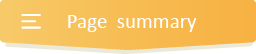## Fun math story problems with answers

Grab the best of very interesting math story problems applicable to real life situations.  These real word math problems have been exceptionally designed to keep your kid’s minds elastic and brilliant in carrying out daily activities.

As a matter of fact, given that word problems are a part of everyday math practice, we have created plenty fun math story problems with answers for each and every math concept.

Most often than not, we strive to encourage kids to think of math problems as actual stories. That is why they’ll find in many areas fun representations, pictures and diagrams, helpful to captivate their interest as well as instruct them on the mathematical relationship found in the word problem.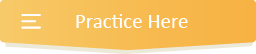2
15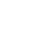243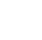3
193934
173695
249663Adding subtracting multiplying and dividing decimals word problems, Adding and subtracting fractions with like denominators word problems, Multiply numbers ending in zeros word problems, Multiplying decimals by whole numbers word problems, Divisibility rules word problems, Dividing whole numbers ending in zeros word problems, Dividing fractions and mixed numbers word problems, Division facts to 12, Estimating products word problems, Adding subtracting multiplying and dividing fractions word problems, Addition and subtraction of whole numbers word problems, Adding and subtracting fractions with unlike denominators word problems, Multiplying by two digit word problems, Multiplying two fractions word problems, Division facts up to 12 word problems, Dividing 2 digit numbers and 3 digit numbers by 2 digit numbers word problems, Divide 2 digit and 3 digit numbers by 2 digit numbers, Estimate sums and differences word problems, Adding subtracting multiplying and dividing whole numbers word problems, Multi step word problems for Grade 5 learners, Adding and subtracting decimals word problems, Multiplying by one digit numbers word problems, Multiplying three or more numbers word problems, Multiplying mixed numbers word problems, Dividing multi digit numbers by 1 digit number word problems, Dividing decimals by whole numbers word problems, Divide multi digit numbers by 1 digit numbers, Estimating quotient word problems, Fractions of whole numbers word problems, Fractions of a number word problems
6
2081153